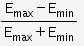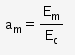# Define the term Modulation index for an AM wave. What would be the modulation

Define the term Modulation index for an AM wave. What would be the modulation index for an AM wave for which the maximum amplitude is ‘a’ while the minimum amplitude id ‘b’?

Modulation index for an AM wave :

The modulation index of an amplitude modulated wave is defined as the ratio of the amplitude of modulating signal (Em) to the amplitude of carrier wave (Ec) i.e.,

Amplitude modulation index, ma =Em/Ec
For a modulated wave,
ma=Em < Ec, to avoid distortion.

M odulation index for an AM wave for which the maximum amplitude is ‘a’ while the minimum amplitude id ‘b’ :

Modulation index,… (1)

Maximum amplitude of modulated wave, a = Ec + Em … (2)
Mimimum amplitude of modulated wave, b = Ec - Em … (3)
From eqn (2) and (3), we get,